Скачать презентацию MGT 3303 Process Analysis Fundamentals Slide 1

4efd8fe289240b07cec1a41140c1def6.ppt

• Количество слайдов: 31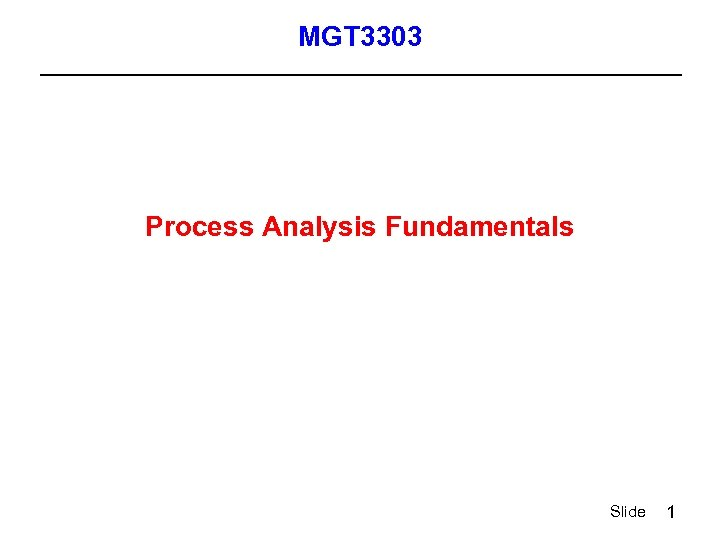MGT 3303 Process Analysis Fundamentals Slide 1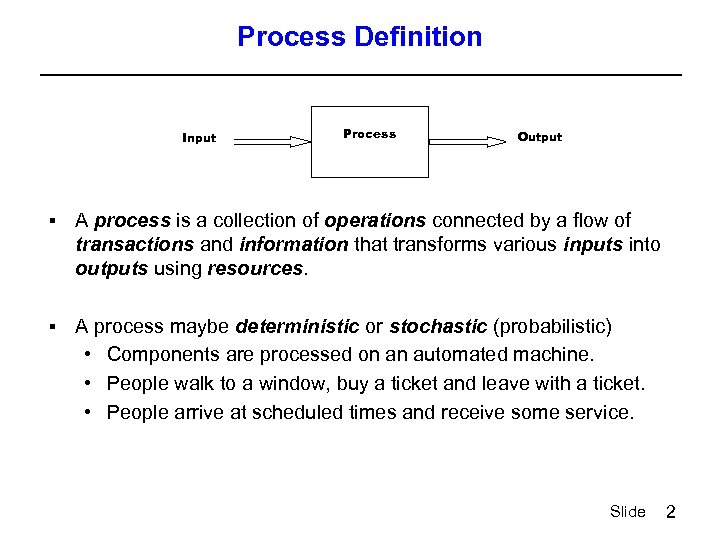Process Definition Input Process Output § A process is a collection of operations connected by a flow of transactions and information that transforms various inputs into outputs using resources. § A process maybe deterministic or stochastic (probabilistic) • Components are processed on an automated machine. • People walk to a window, buy a ticket and leave with a ticket. • People arrive at scheduled times and receive some service. Slide 2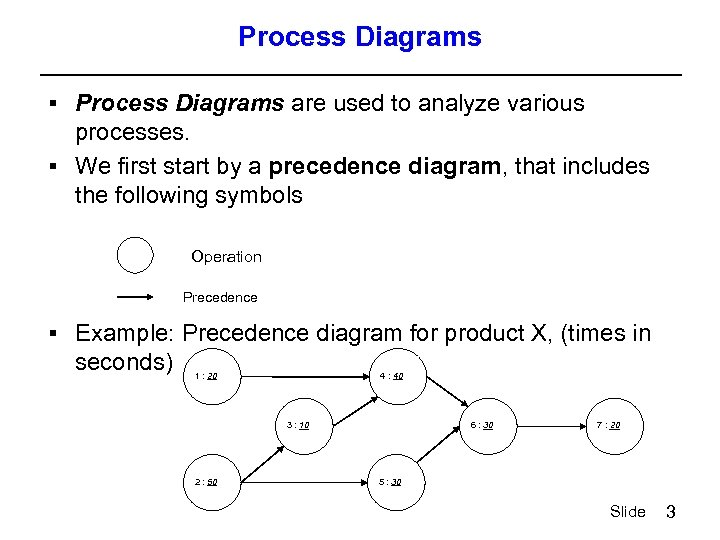Process Diagrams § Process Diagrams are used to analyze various processes. § We first start by a precedence diagram, that includes the following symbols Operation Precedence § Example: Precedence diagram for product X, (times in seconds) 1 : 20 4 : 40 3 : 10 2 : 50 6 : 30 5 : 30 7 : 20 Slide 3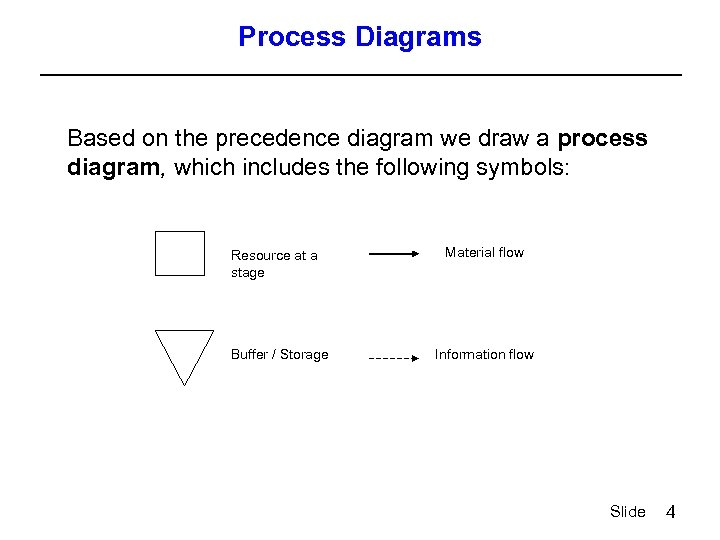Process Diagrams Based on the precedence diagram we draw a process diagram, which includes the following symbols: Resource at a stage Material flow Buffer / Storage Information flow Slide 4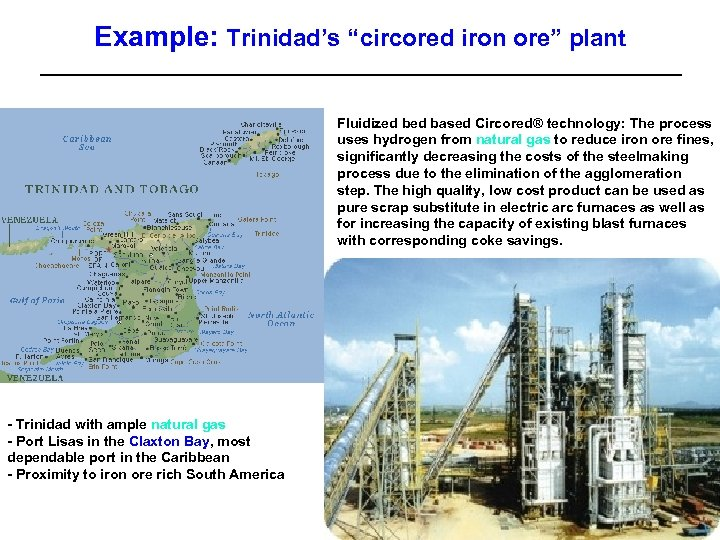Example: Trinidad’s “circored iron ore” plant Fluidized based Circored® technology: The process uses hydrogen from natural gas to reduce iron ore fines, significantly decreasing the costs of the steelmaking process due to the elimination of the agglomeration step. The high quality, low cost product can be used as pure scrap substitute in electric arc furnaces as well as for increasing the capacity of existing blast furnaces with corresponding coke savings. - Trinidad with ample natural gas - Port Lisas in the Claxton Bay, most dependable port in the Caribbean - Proximity to iron ore rich South America Slide 5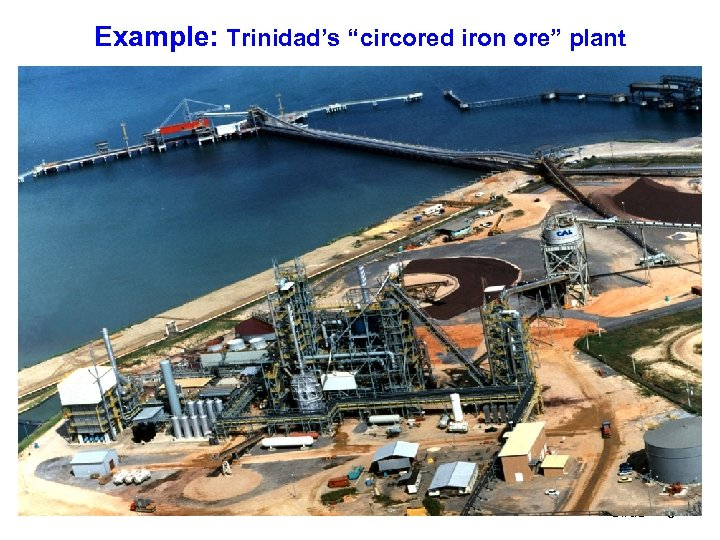Example: Trinidad’s “circored iron ore” plant Slide 6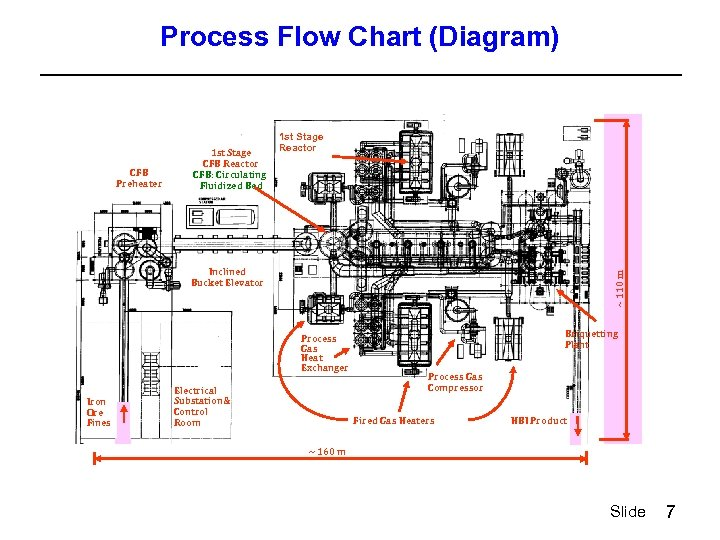Process Flow Chart (Diagram) CFB Preheater 1 st Stage CFB Reactor CFB: Circulating Fluidized Bed 1 st Stage Reactor ~ 110 m Inclined Bucket Elevator Process Gas Heat Exchanger Iron Ore Fines Electrical Substation& Control Room Briquetting Plant Process Gas Compressor Fired Gas Heaters HBI Product ~ 160 m Slide 7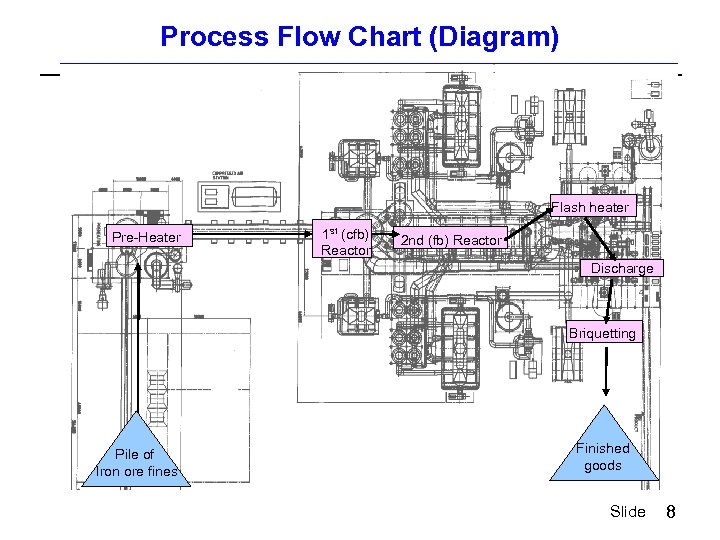Process Flow Chart (Diagram) Flash heater Pre-Heater 1 st (cfb) Reactor 2 nd (fb) Reactor Discharge Briquetting Pile of Iron ore fines Finished goods Slide 8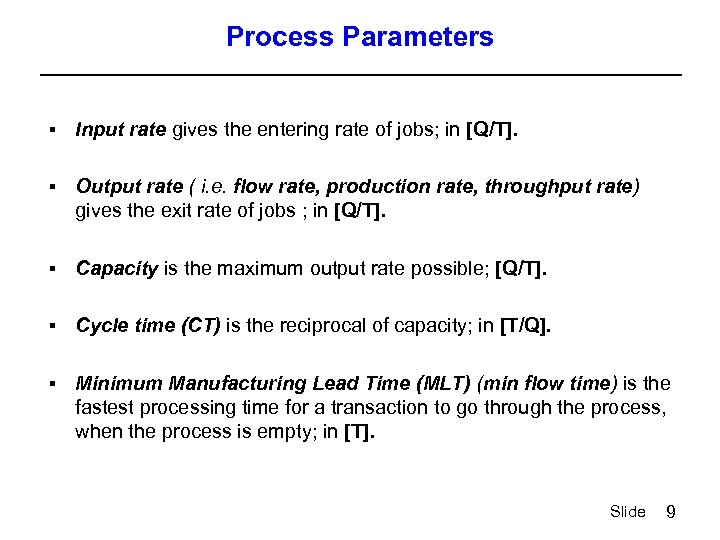Process Parameters § Input rate gives the entering rate of jobs; in [Q/T]. § Output rate ( i. e. flow rate, production rate, throughput rate) gives the exit rate of jobs ; in [Q/T]. § Capacity is the maximum output rate possible; [Q/T]. § Cycle time (CT) is the reciprocal of capacity; in [T/Q]. § Minimum Manufacturing Lead Time (MLT) (min flow time) is the fastest processing time for a transaction to go through the process, when the process is empty; in [T]. Slide 9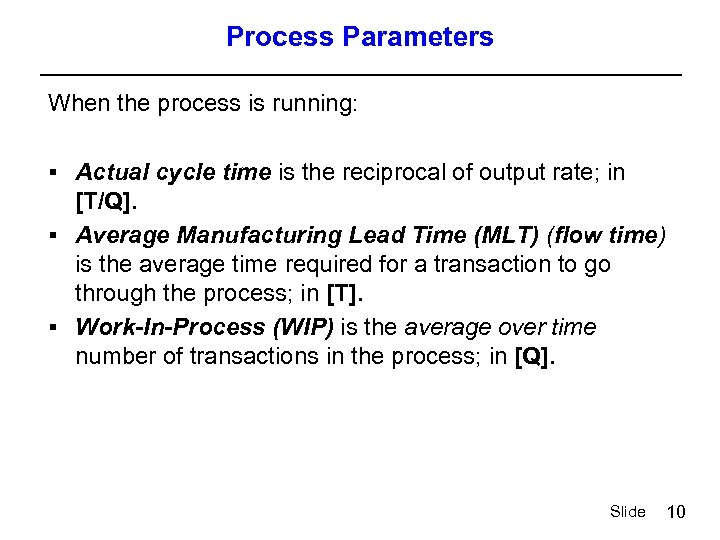Process Parameters When the process is running: § Actual cycle time is the reciprocal of output rate; in [T/Q]. § Average Manufacturing Lead Time (MLT) (flow time) is the average time required for a transaction to go through the process; in [T]. § Work-In-Process (WIP) is the average over time number of transactions in the process; in [Q]. Slide 10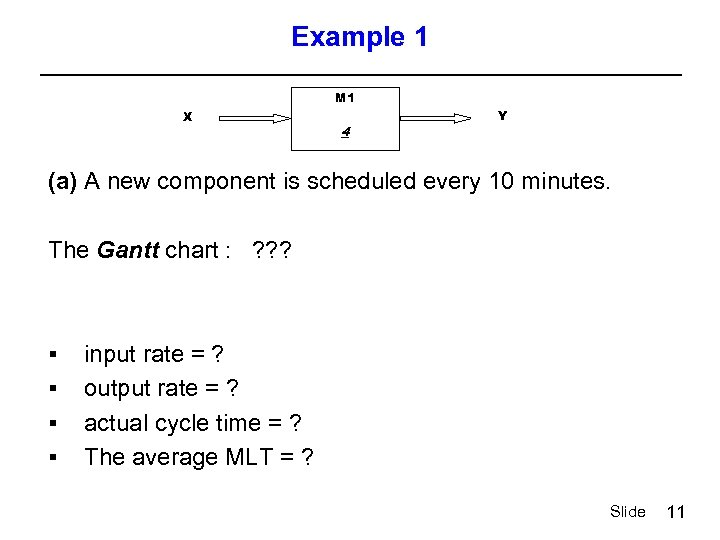Example 1 M 1 X 4 Y (a) A new component is scheduled every 10 minutes. The Gantt chart : ? ? ? § § input rate = ? output rate = ? actual cycle time = ? The average MLT = ? Slide 11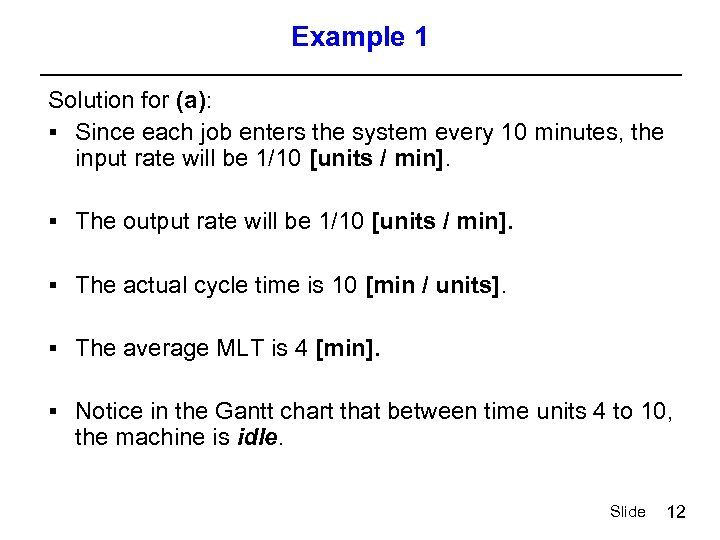Example 1 Solution for (a): § Since each job enters the system every 10 minutes, the input rate will be 1/10 [units / min]. § The output rate will be 1/10 [units / min]. § The actual cycle time is 10 [min / units]. § The average MLT is 4 [min]. § Notice in the Gantt chart that between time units 4 to 10, the machine is idle. Slide 12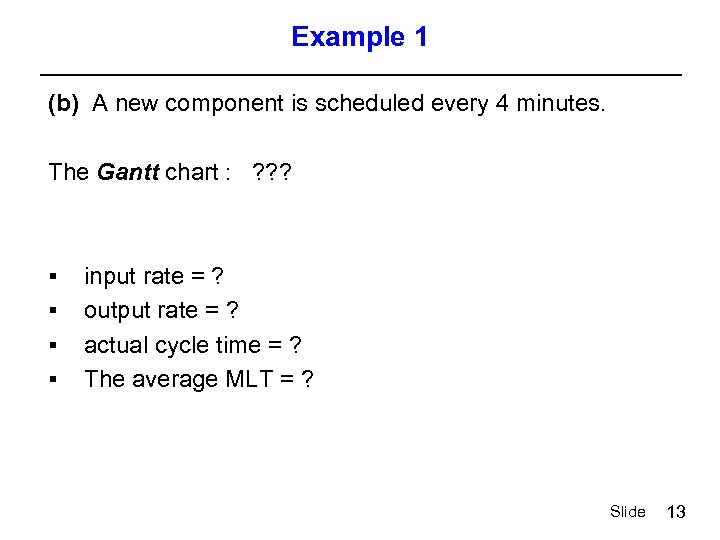Example 1 (b) A new component is scheduled every 4 minutes. The Gantt chart : ? ? ? § § input rate = ? output rate = ? actual cycle time = ? The average MLT = ? Slide 13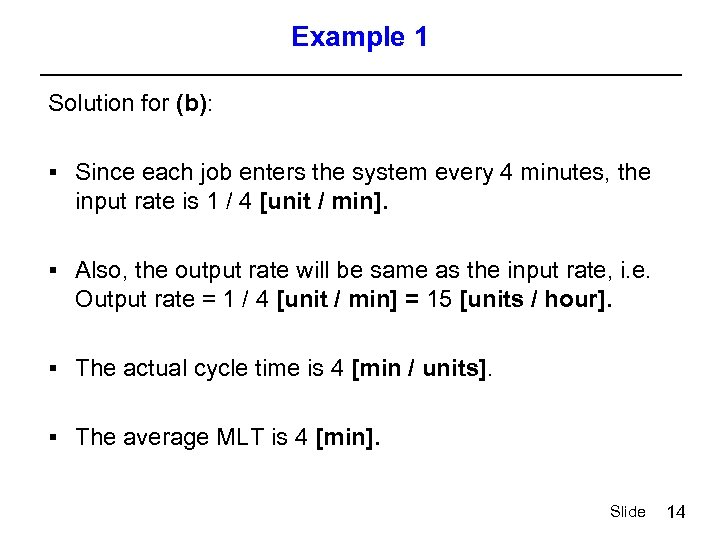Example 1 Solution for (b): § Since each job enters the system every 4 minutes, the input rate is 1 / 4 [unit / min]. § Also, the output rate will be same as the input rate, i. e. Output rate = 1 / 4 [unit / min] = 15 [units / hour]. § The actual cycle time is 4 [min / units]. § The average MLT is 4 [min]. Slide 14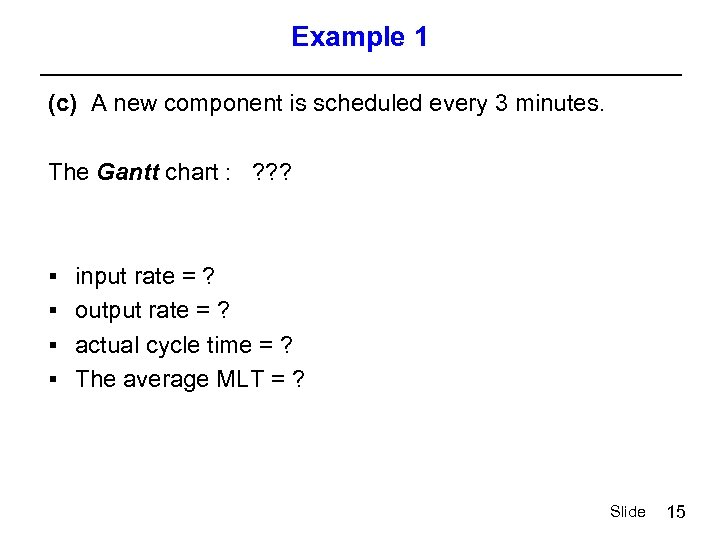Example 1 (c) A new component is scheduled every 3 minutes. The Gantt chart : ? ? ? § input rate = ? § output rate = ? § actual cycle time = ? § The average MLT = ? Slide 15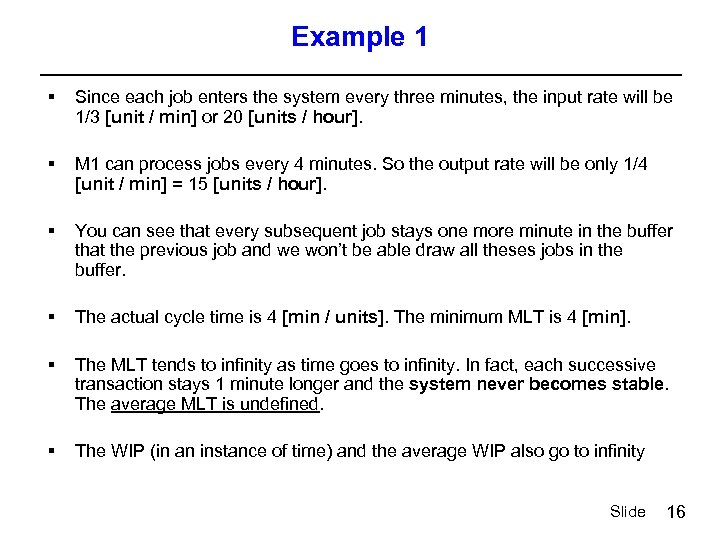Example 1 § Since each job enters the system every three minutes, the input rate will be 1/3 [unit / min] or 20 [units / hour]. § M 1 can process jobs every 4 minutes. So the output rate will be only 1/4 [unit / min] = 15 [units / hour]. § You can see that every subsequent job stays one more minute in the buffer that the previous job and we won’t be able draw all theses jobs in the buffer. § The actual cycle time is 4 [min / units]. The minimum MLT is 4 [min]. § The MLT tends to infinity as time goes to infinity. In fact, each successive transaction stays 1 minute longer and the system never becomes stable. The average MLT is undefined. § The WIP (in an instance of time) and the average WIP also go to infinity Slide 16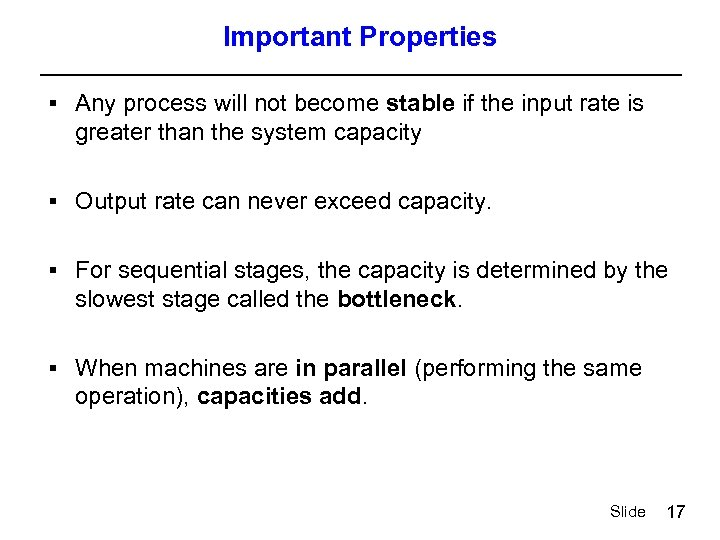Important Properties § Any process will not become stable if the input rate is greater than the system capacity § Output rate can never exceed capacity. § For sequential stages, the capacity is determined by the slowest stage called the bottleneck. § When machines are in parallel (performing the same operation), capacities add. Slide 17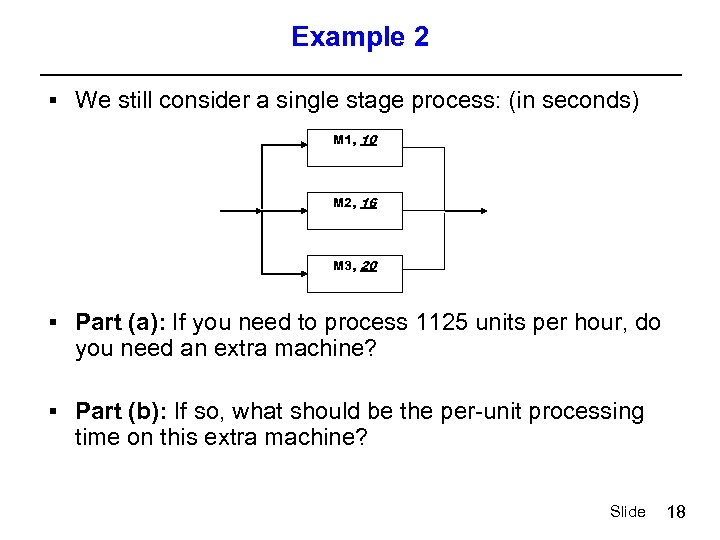Example 2 § We still consider a single stage process: (in seconds) M 1, 10 M 2, 16 M 3, 20 § Part (a): If you need to process 1125 units per hour, do you need an extra machine? § Part (b): If so, what should be the per-unit processing time on this extra machine? Slide 18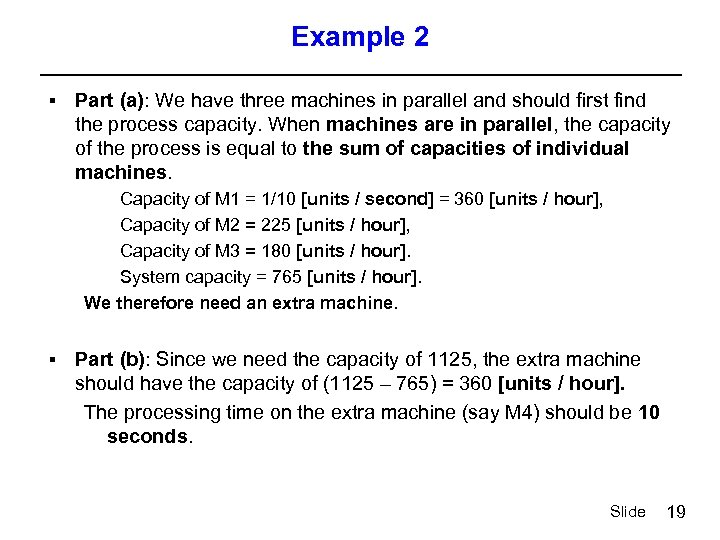Example 2 § Part (a): We have three machines in parallel and should first find the process capacity. When machines are in parallel, the capacity of the process is equal to the sum of capacities of individual machines. Capacity of M 1 = 1/10 [units / second] = 360 [units / hour], Capacity of M 2 = 225 [units / hour], Capacity of M 3 = 180 [units / hour]. System capacity = 765 [units / hour]. We therefore need an extra machine. § Part (b): Since we need the capacity of 1125, the extra machine should have the capacity of (1125 – 765) = 360 [units / hour]. The processing time on the extra machine (say M 4) should be 10 seconds. Slide 19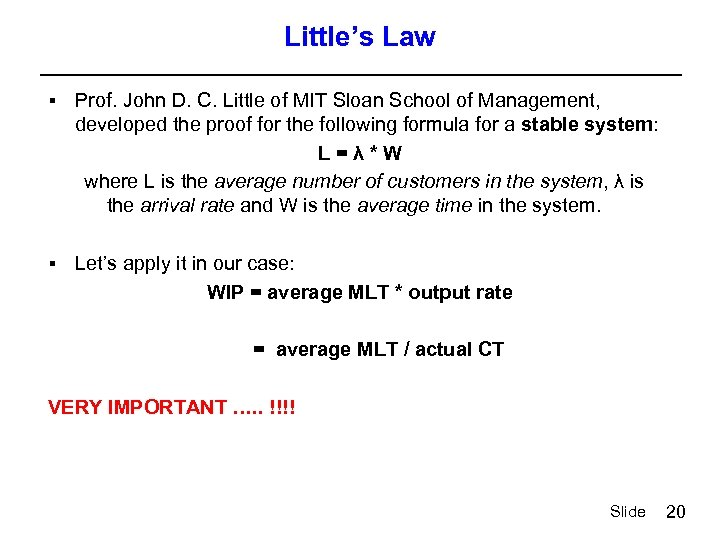Little’s Law § Prof. John D. C. Little of MIT Sloan School of Management, developed the proof for the following formula for a stable system: L=λ*W where L is the average number of customers in the system, λ is the arrival rate and W is the average time in the system. § Let’s apply it in our case: WIP = average MLT * output rate = average MLT / actual CT VERY IMPORTANT …. . !!!! Slide 20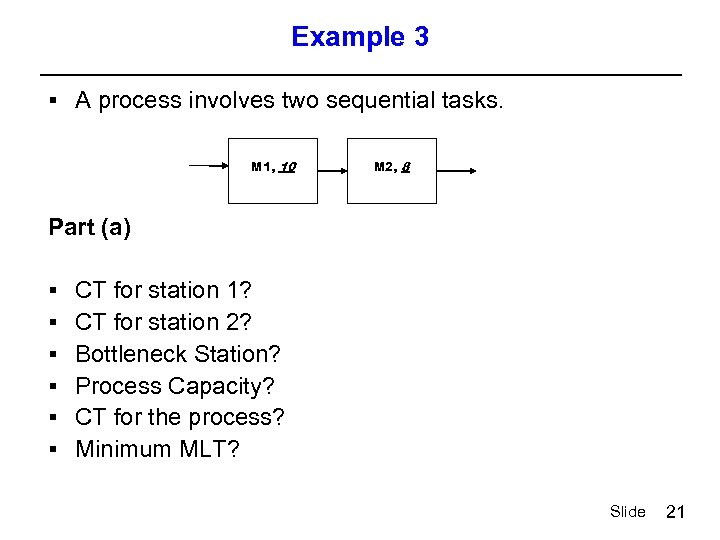Example 3 § A process involves two sequential tasks. M 1, 10 M 2, 8 Part (a) § § § CT for station 1? CT for station 2? Bottleneck Station? Process Capacity? CT for the process? Minimum MLT? Slide 21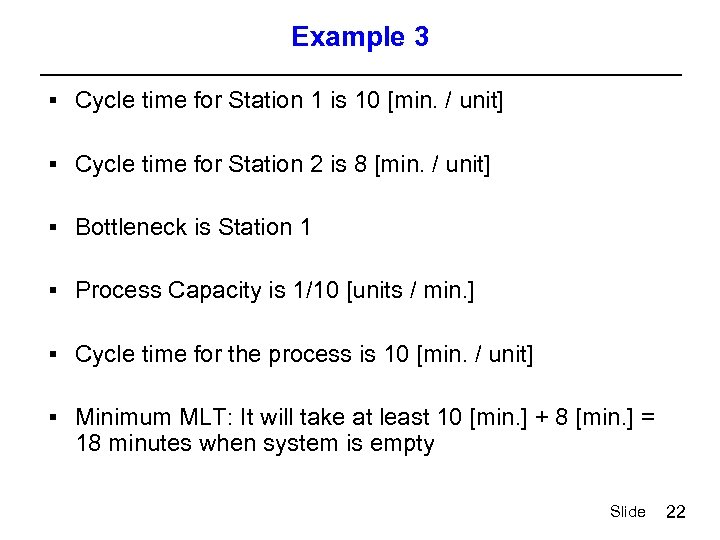Example 3 § Cycle time for Station 1 is 10 [min. / unit] § Cycle time for Station 2 is 8 [min. / unit] § Bottleneck is Station 1 § Process Capacity is 1/10 [units / min. ] § Cycle time for the process is 10 [min. / unit] § Minimum MLT: It will take at least 10 [min. ] + 8 [min. ] = 18 minutes when system is empty Slide 22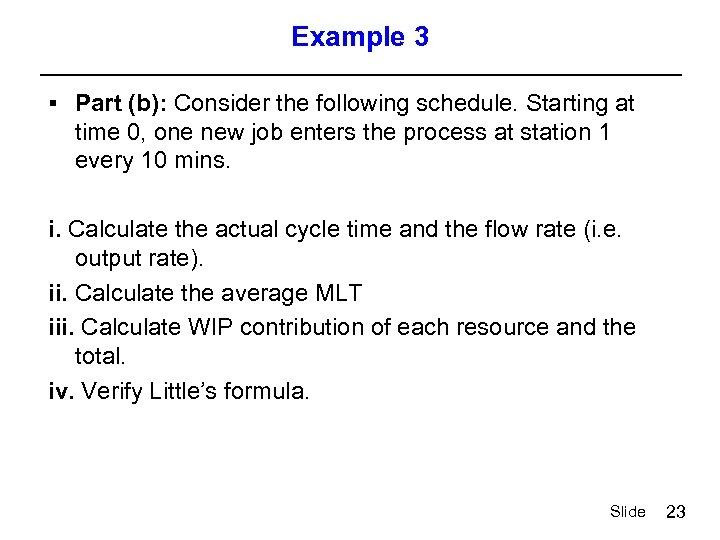Example 3 § Part (b): Consider the following schedule. Starting at time 0, one new job enters the process at station 1 every 10 mins. i. Calculate the actual cycle time and the flow rate (i. e. output rate). ii. Calculate the average MLT iii. Calculate WIP contribution of each resource and the total. iv. Verify Little’s formula. Slide 23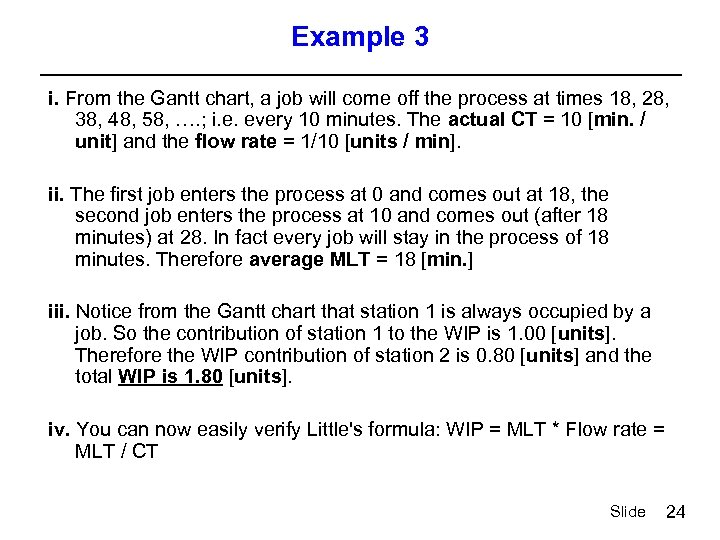Example 3 i. From the Gantt chart, a job will come off the process at times 18, 28, 38, 48, 58, …. ; i. e. every 10 minutes. The actual CT = 10 [min. / unit] and the flow rate = 1/10 [units / min]. ii. The first job enters the process at 0 and comes out at 18, the second job enters the process at 10 and comes out (after 18 minutes) at 28. In fact every job will stay in the process of 18 minutes. Therefore average MLT = 18 [min. ] iii. Notice from the Gantt chart that station 1 is always occupied by a job. So the contribution of station 1 to the WIP is 1. 00 [units]. Therefore the WIP contribution of station 2 is 0. 80 [units] and the total WIP is 1. 80 [units]. iv. You can now easily verify Little's formula: WIP = MLT * Flow rate = MLT / CT Slide 24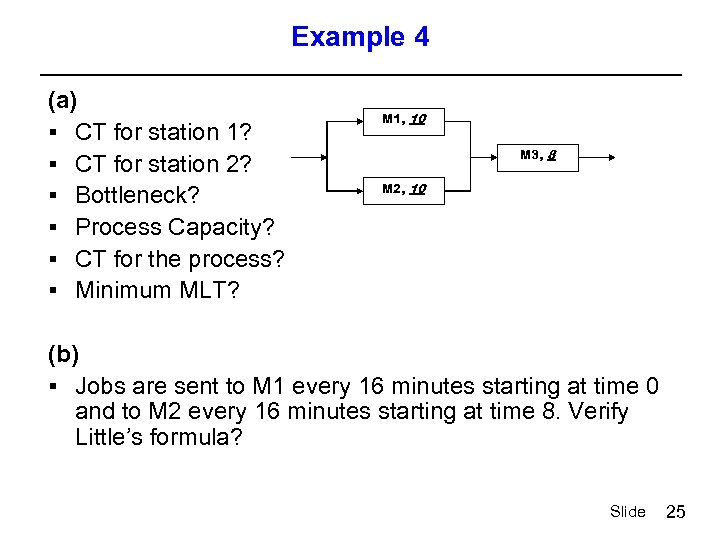Example 4 (a) § CT for station 1? § CT for station 2? § Bottleneck? § Process Capacity? § CT for the process? § Minimum MLT? M 1, 10 M 3, 8 M 2, 10 (b) § Jobs are sent to M 1 every 16 minutes starting at time 0 and to M 2 every 16 minutes starting at time 8. Verify Little’s formula? Slide 25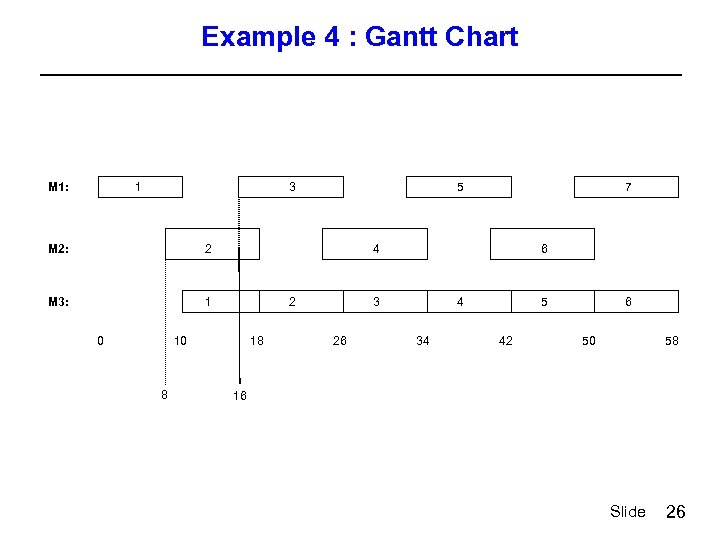Example 4 : Gantt Chart M 1: 1 M 2: 2 1 3 M 3: 2 4 3 5 4 6 5 7 6 0 10 18 26 34 42 50 58 8 16 Slide 26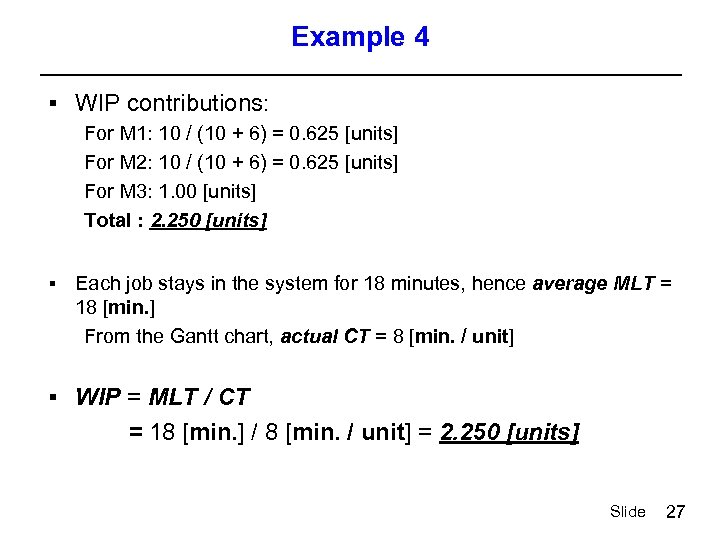Example 4 § WIP contributions: For M 1: 10 / (10 + 6) = 0. 625 [units] For M 2: 10 / (10 + 6) = 0. 625 [units] For M 3: 1. 00 [units] Total : 2. 250 [units] § Each job stays in the system for 18 minutes, hence average MLT = 18 [min. ] From the Gantt chart, actual CT = 8 [min. / unit] § WIP = MLT / CT = 18 [min. ] / 8 [min. / unit] = 2. 250 [units] Slide 27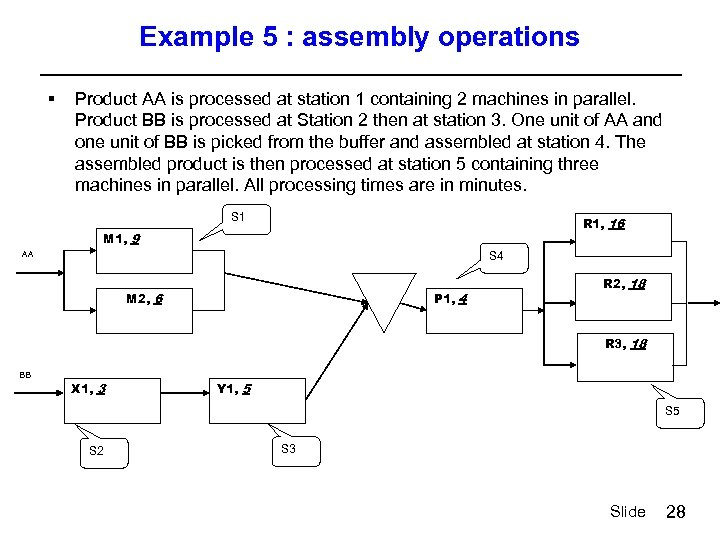Example 5 : assembly operations § Product AA is processed at station 1 containing 2 machines in parallel. Product BB is processed at Station 2 then at station 3. One unit of AA and one unit of BB is picked from the buffer and assembled at station 4. The assembled product is then processed at station 5 containing three machines in parallel. All processing times are in minutes. S 1 R 1, 16 M 1, 9 S 4 AA M 2, 6 P 1, 4 R 2, 18 R 3, 18 BB X 1, 3 Y 1, 5 S 2 S 3 Slide 28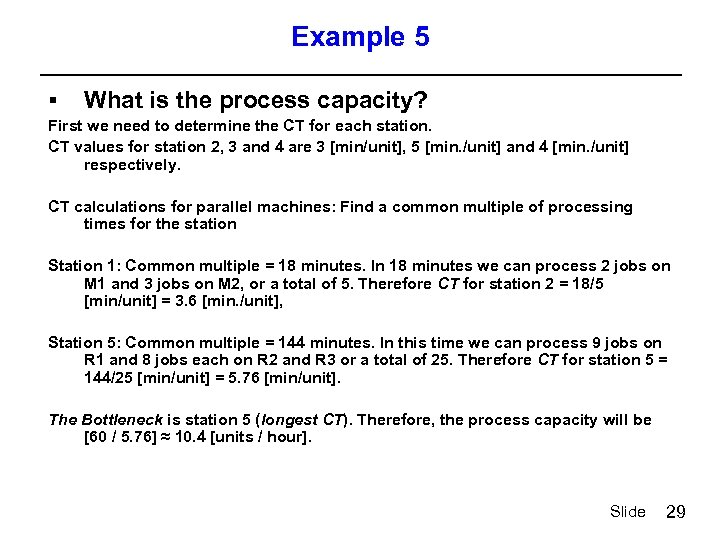Example 5 § What is the process capacity? First we need to determine the CT for each station. CT values for station 2, 3 and 4 are 3 [min/unit], 5 [min. /unit] and 4 [min. /unit] respectively. CT calculations for parallel machines: Find a common multiple of processing times for the station Station 1: Common multiple = 18 minutes. In 18 minutes we can process 2 jobs on M 1 and 3 jobs on M 2, or a total of 5. Therefore CT for station 2 = 18/5 [min/unit] = 3. 6 [min. /unit], Station 5: Common multiple = 144 minutes. In this time we can process 9 jobs on R 1 and 8 jobs each on R 2 and R 3 or a total of 25. Therefore CT for station 5 = 144/25 [min/unit] = 5. 76 [min/unit]. The Bottleneck is station 5 (longest CT). Therefore, the process capacity will be [60 / 5. 76] ≈ 10. 4 [units / hour]. Slide 29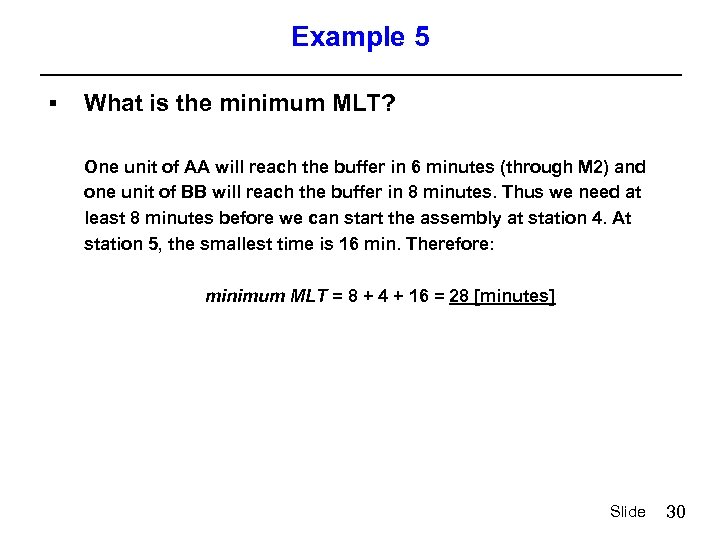Example 5 § What is the minimum MLT? One unit of AA will reach the buffer in 6 minutes (through M 2) and one unit of BB will reach the buffer in 8 minutes. Thus we need at least 8 minutes before we can start the assembly at station 4. At station 5, the smallest time is 16 min. Therefore: minimum MLT = 8 + 4 + 16 = 28 [minutes] Slide 30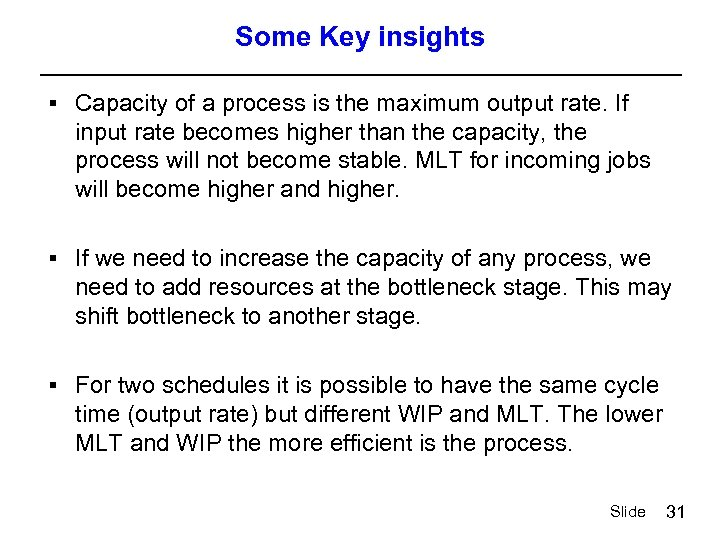Some Key insights § Capacity of a process is the maximum output rate. If input rate becomes higher than the capacity, the process will not become stable. MLT for incoming jobs will become higher and higher. § If we need to increase the capacity of any process, we need to add resources at the bottleneck stage. This may shift bottleneck to another stage. § For two schedules it is possible to have the same cycle time (output rate) but different WIP and MLT. The lower MLT and WIP the more efficient is the process. Slide 31